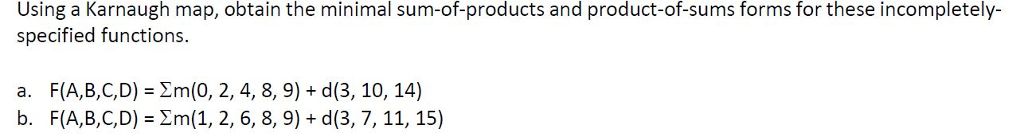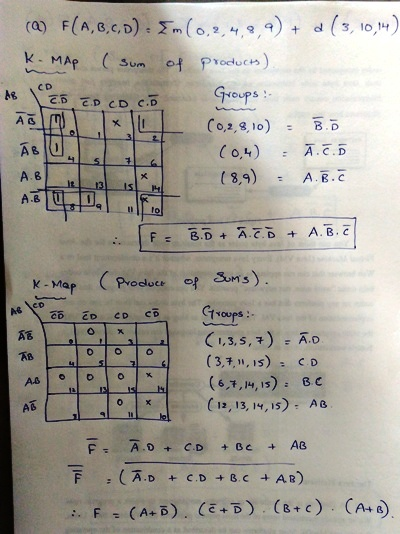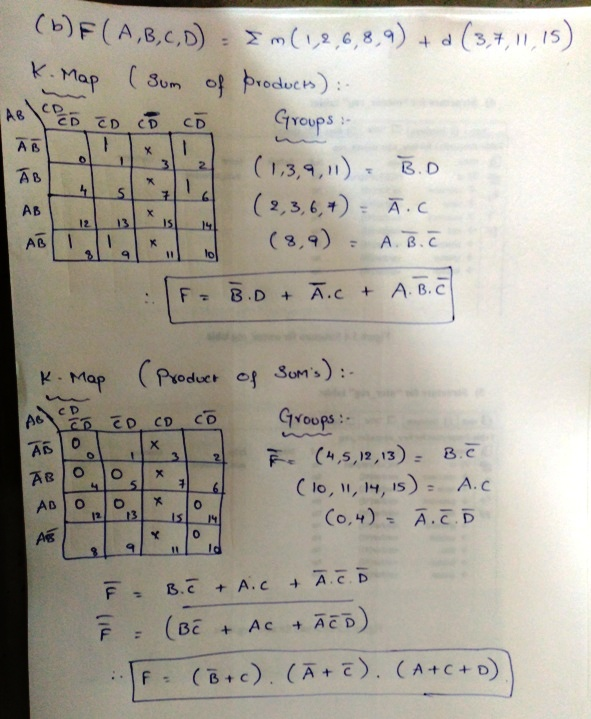# Answered! Using a Karnaugh map, obtain the minimal sum-of-products and product-of-sums forms for these incompletely-…Using a Karnaugh map, obtain the minimal sum-of-products and product-of-sums forms for these incompletely-specified functions. a. F(A, B, C, D) = sigma m(0, 2, 4, 8, 9) + d(3, 10, 14) b. F(A, B, C, D) = sigma m(l, 2, 6, 8, 9) + d(3, 7, 11, 15)